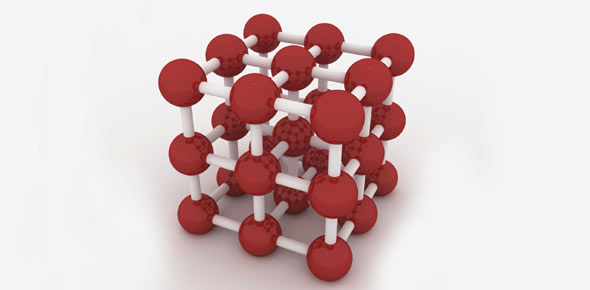# Is Atoms Your Forte? Find Out With This Quiz!

14 Questions | Total Attempts: 94SettingsDo you think that you know all there is about atoms such as the findings, changes and breakthroughs that came with understanding them? If you said yes then this is your chance to put your answer to the test. Take up this quiz and share your score in the comment section.

• 1.
An atom composed of 16 protons, 16 electrons, and 16 neutrons is
• A.

Sulfur-48

• B.

Germanium-32

• C.

Sulfur-32

• D.

Sulfur-16

• 2.
Which of these descriptions is incorrect?
• A.

Proton: positive charge, in nucleus, mass of 1

• B.

Electron: negative charge, mass of 0, in nucleus

• C.

Neutron: mass of 1, no charge

• 3.
Thallium has two isotopes, thallium-203 adn thallium-205. Thallium's atomic number is 81 and its atomic mass is 204.38 amu. Which statement about the thallium isotopes is true?
• A.

There is more thallium-203 in nature

• B.

Atoms of both isotopes have 81 protons

• C.

Thallium-205 atoms have fewer neutrons

• D.

The most common atom of thallium has a mass of 204.38 amu

• 4.
Every aluminum-27 atom has 27 protons and 27 electrons.
• A.

True

• B.

False

• 5.
Isotopes of an element have different masses.
• A.

True

• B.

False

• 6.
A weighted average counts every number equally.
• A.

True

• B.

False

• 7.
Which scientist contributed the discovery of the dense central nucleus in the atom,  surrounded by primarily empty space?
• A.

JJ Thompson

• B.

Ernest Rutherford

• C.

Neils Bohr

• D.

Democritus

• 8.
Choose all of the following that represent subatomic particles:
• A.

Protons

• B.

Neutrons

• C.

Electrons

• D.

Nucleus

• 9.
Calcium has an atomic number of 20. The mass of a calcium isotope is 40. How many neutrons does calcium have? Answer using the number ONLY.
• 10.
• A.

204

• B.

206

• C.

207

• D.

208

• 11.
• A.

208

• B.

82

• C.

52

• D.

208-82

• 12.
The four isotopes of lead are   lead-204 (1.4%), lead-206 (24.1%), lead-207 (22.1%), and lead-208 (52.4%). Which of the following represents the average atomic mass based on the information given?
• A.

207.24

• B.

206.25

• C.

208

• D.

205.67

• 13.
Imagine you are standing on the top of a boron-11 nucleus. Describe the numbers and kinds of subatomic particles you would see looking down into the nucleus, and those you would see looking out from the nucleus. (boron is element number 5)
• 14.
If you know the atomic number and mass number of an atom of an element, how can you determine the number of protons, neutrons, and electrons in that atom?
Related TopicsBack to top